# 工字形钢梁构件有限元模拟一般性能分析研究General Performance Analysis of Finite Element Simulation of I-Shaped Steel Beam Members

DOI: 10.12677/HJCE.2019.89155, PDF, HTML, XML, 下载: 253  浏览: 462

Abstract: Taking the simply supported I-shaped steel beam as an example, the general stress characteristics of the I-shaped steel beam under ABAQUS finite element simulation software are introduced. One is the relationship between stress-strain and the change with time under external load relationship. The other is the relationship between deflection and displacement with time under external load to comprehensively determine the force change and deformation development of the steel beam under uniform load.

1. 概述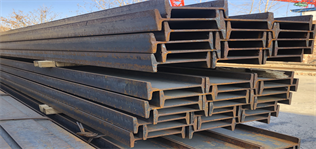Figure 1. Schematic diagram of I-beam steel beam

2. 有限元分析模型

2.1. 有限元建模设计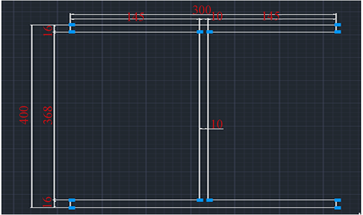Figure 2. Schematic diagram of cross-section dimensions of I-shaped steel beams

2.2. 有限元建模分析过程

$\eta$ 值一般取在1.05以内，刚度不够是可以调整工字形钢梁的截面尺寸，例如：可以增加截面的有效高度 。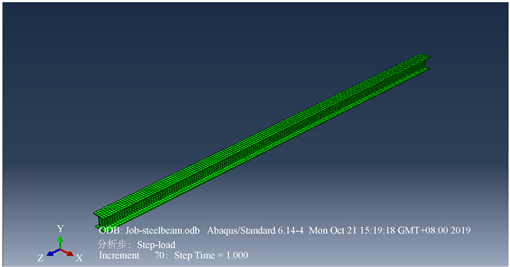Figure 3. Finite element simulation undeformed graph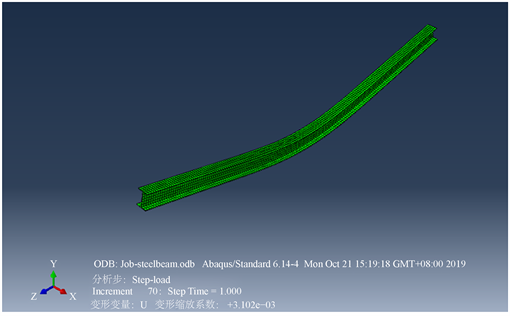Figure 4. Finite element simulation deformation map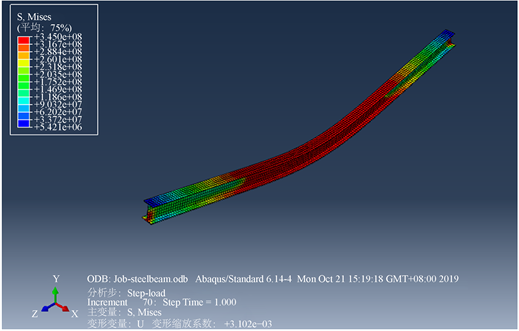Figure 5. Deformation map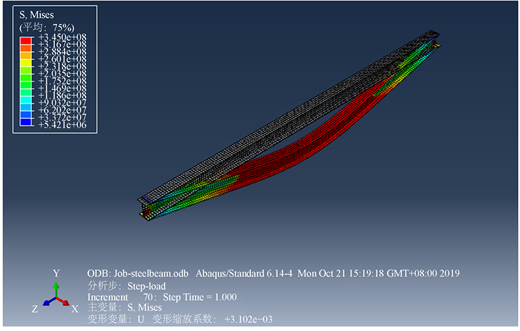Figure 6. Unbalanced and deformed graphs comparing stress clouds

${\sigma }_{cr}=\frac{{M}_{cr}}{{W}_{x}},\text{\hspace{0.17em}}\text{\hspace{0.17em}}{\phi }_{b}=\frac{{\sigma }_{cr}}{{f}_{y}}=\frac{{M}_{cr}}{{W}_{x}{f}_{y}}$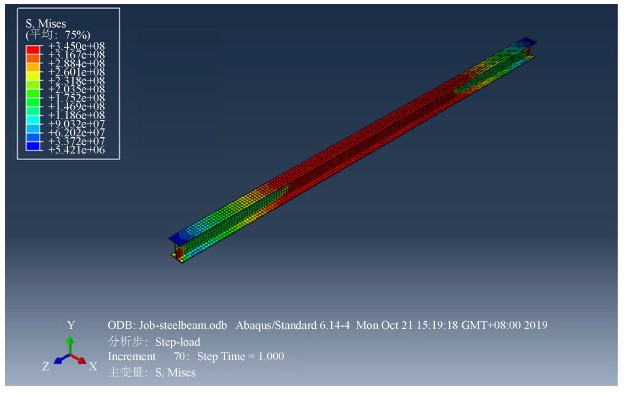Figure 7. Stress cloud on undeformed graph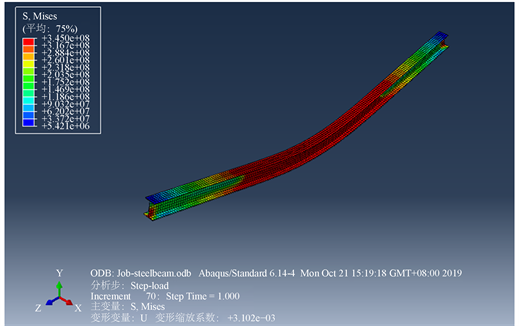Figure 8. Drawing a stress cloud on a deformed graph

${\phi }_{b}={\beta }_{b}\frac{4320}{{\lambda }_{y}^{2}}\frac{Ah}{{W}_{x}}\left[\sqrt{1+{\left(\frac{{\lambda }_{y}{t}_{1}}{4.4h}\right)}^{2}}+{\eta }_{b}\right]\frac{235}{{f}_{y}}$

2.3. 有限元建模结果分析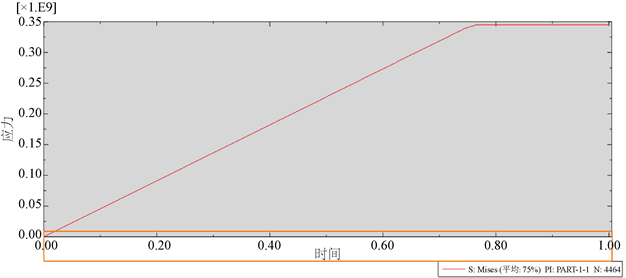Figure 9. Stress versus time curve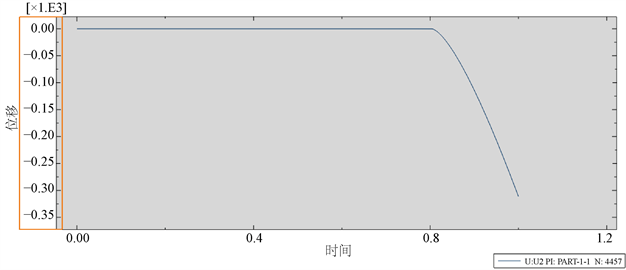Figure 10. Displacement versus time curve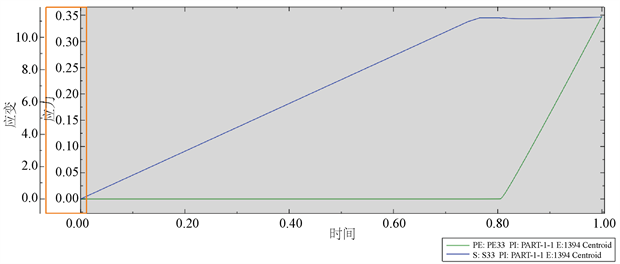Figure 11. Stress-strain versus time curve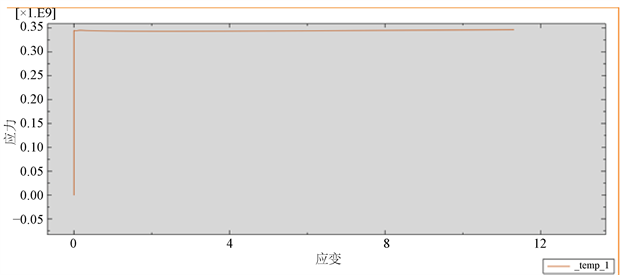Figure 12. Stress-strain relationship curve

  中华人民共和国住房与城乡建设部. 钢结构设计规范GB50017-2017 [S]. 北京: 中国计划出版社, 2017.  王言磊, 李芦钰, 侯吉林, 安永辉. 土木工程常用软件与应用[M]. 北京: 中国建筑工业出版社, 2017.  赵根田, 孙德发. 钢结构[M]. 北京: 机械工业出版社, 2010.  曹平周, 朱召全. 钢结构[M]. 北京: 中国电力出版社, 2015.  牛秀艳, 刘伟. 钢结构基本原理[M]. 武汉: 武汉大学出版社, 2018.  沈祖炎, 陈扬骥, 陈以一. 钢结构基本原理[M]. 北京: 中国建筑工业出版社, 2005.  中华人民共和国住房与城乡建设部. 型钢轧钢工程设计规范GB50410-2014 [S]. 北京: 中国计划出版社, 2014.  中华人民共和国住房与城乡建设部. 小型型钢轧钢工艺设计规范GB50410-2007 [S]. 北京: 中国计划出版社, 2007.